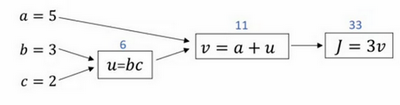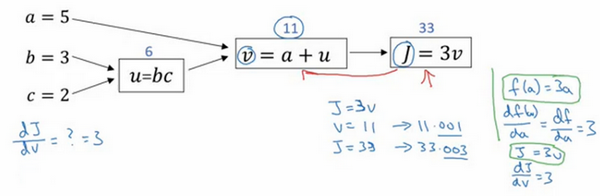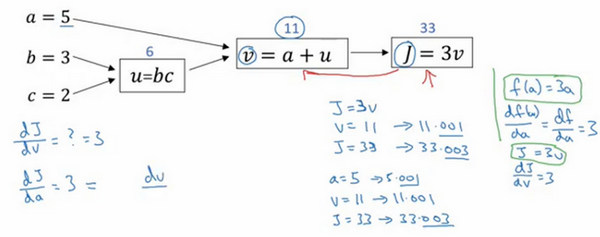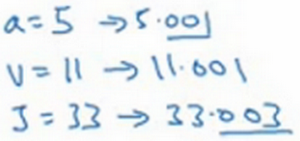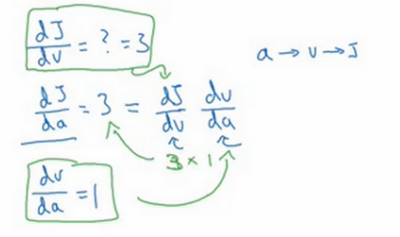## 第二周：神经网络的编程基础(Basics of Neural Network programming)

### 2.1 二分类(Binary Classification)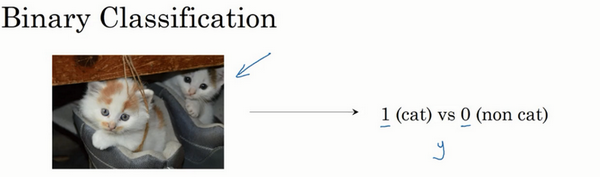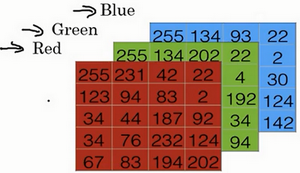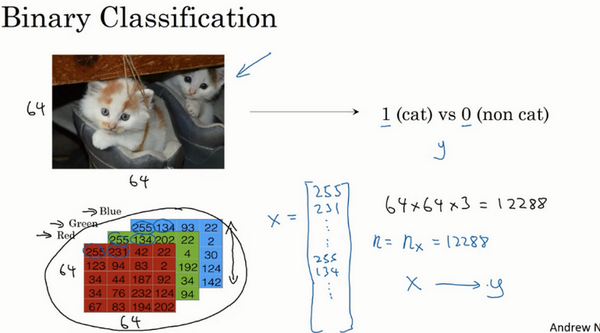$x$：表示一个$n_x$维数据，为输入数据，维度为$(n_x,1)$；

$y$：表示输出结果，取值为$(0,1)$

$(x^{(i)},y^{(i)})$：表示第$i$组数据，可能是训练数据，也可能是测试数据，此处默认为训练数据；

$X=[x^{(1)},x^{(2)},...,x^{(m)}]$：表示所有的训练数据集的输入值，放在一个 $n_x×m$的矩阵中，其中$m$表示样本数目;

$Y=[y^{(1)},y^{(2)},...,y^{(m)}]$：对应表示所有训练数据集的输出值，维度为$1×m$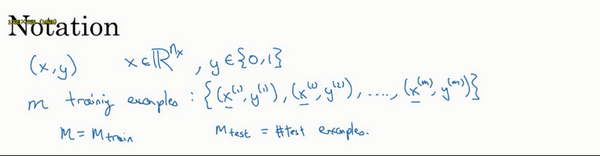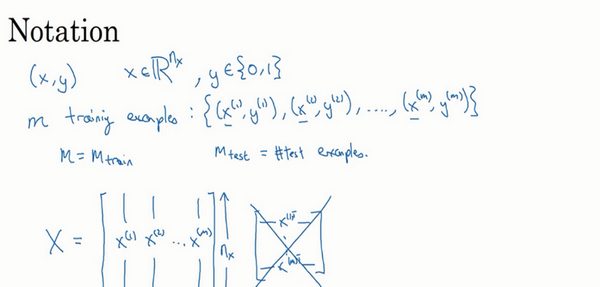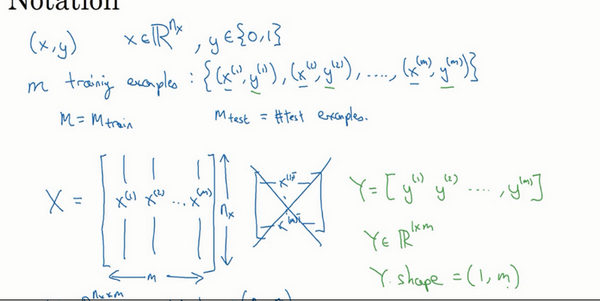### 2.2 逻辑回归(Logistic Regression)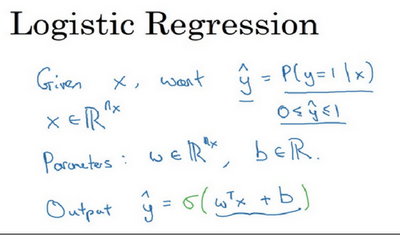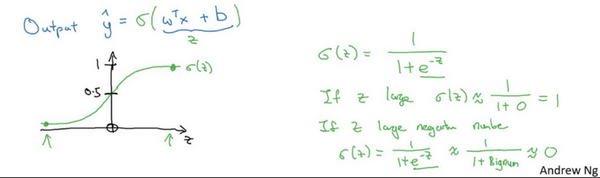### 2.3 逻辑回归的代价函数（Logistic Regression Cost Function）$y=1$时损失函数$L=-\log (\hat{y})$，如果想要损失函数$L$尽可能得小，那么$\hat{y}$就要尽可能大，因为sigmoid函数取值$[0,1]$，所以$\hat{y}$会无限接近于1。

$y=0$时损失函数$L=-\log (1-\hat{y})$，如果想要损失函数$L$尽可能得小，那么$\hat{y}$就要尽可能小，因为sigmoid函数取值$[0,1]$，所以$\hat{y}$会无限接近于0。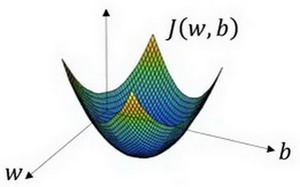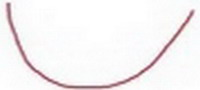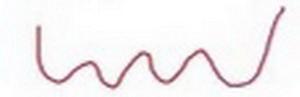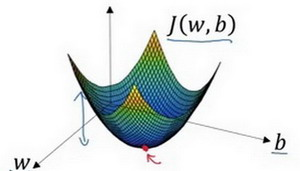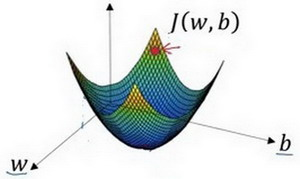2. 朝最陡的下坡方向走一步，不断地迭代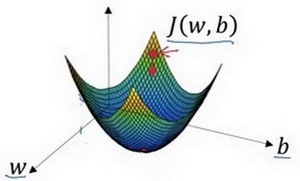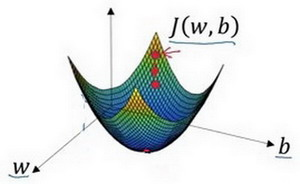3.直到走到全局最优解或者接近全局最优解的地方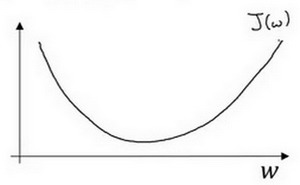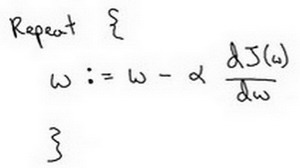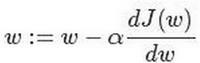$:=$表示更新参数,

$a$ 表示学习率（learning rate），用来控制步长（step），即向下走一步的长度$\frac{dJ(w)}{dw}$ 就是函数$J(w)$$w$ 求导（derivative），在代码中我们会使用$dw$表示这个结果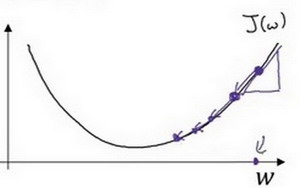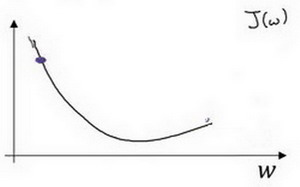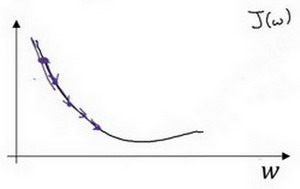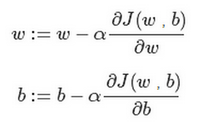$\partial$ 表示求偏导符号，可以读作round$\frac{\partial J(w,b)}{\partial w}$ 就是函数$J(w,b)$$w$ 求偏导，在代码中我们会使用$dw$ 表示这个结果， $\frac{\partial J(w,b)}{\partial b}$ 就是函数$J(w,b)$$b$ 求偏导，在代码中我们会使用$db$ 表示这个结果， 小写字母$d$ 用在求导数（derivative），即函数只有一个参数， 偏导数符号$\partial$ 用在求偏导（partial derivative），即函数含有两个以上的参数。

### 2.5 导数（Derivatives）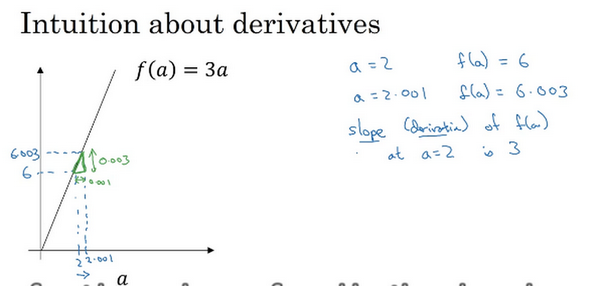### 2.6 更多的导数例子（More Derivative Examples）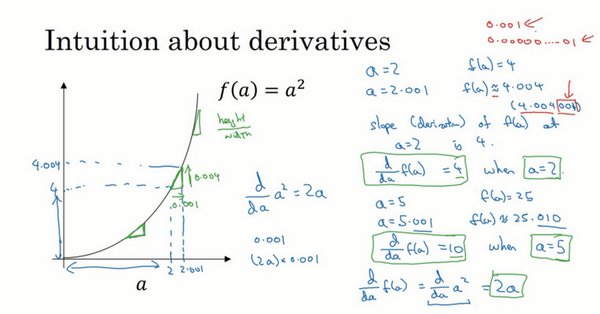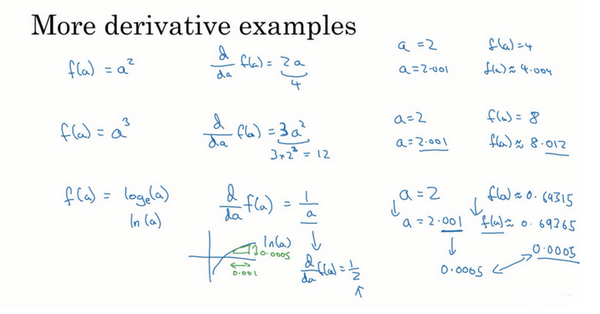### 2.7 计算图（Computation Graph）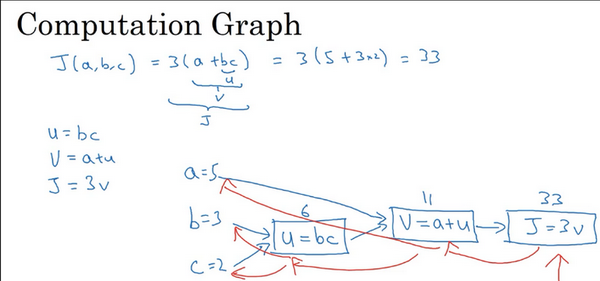### 2.8 使用计算图求导数（Derivatives with a Computation Graph）

$\frac{dJ}{du}=\frac{dJ}{dv}\frac{dv}{du}$$\frac{dJ}{db}=\frac{dJ}{du}\frac{du}{db}$$\frac{dJ}{da}=\frac{dJ}{du}\frac{du}{da}$